# MySQL

And and Or in MySQL
 Previous Home Next

### And

This can be used as dependently with where clause commands. And keyword can be used where we need to satisfy more than one condition or atleast two conditions(options) simultaneously need to satisfy within a command to get the desired output. Syntax of And with Where clause

```SELECT expressions
FROM tables
WHERE columnname optionalvalue1
AND column optionalvalue2;
```

so lets see some examples given below while using the previous database and its tables.

### Example

```SELECT * 					//show all values
WHERE  country='India' 				//AND condition is given
AND locatn ='central' ;
```

### output become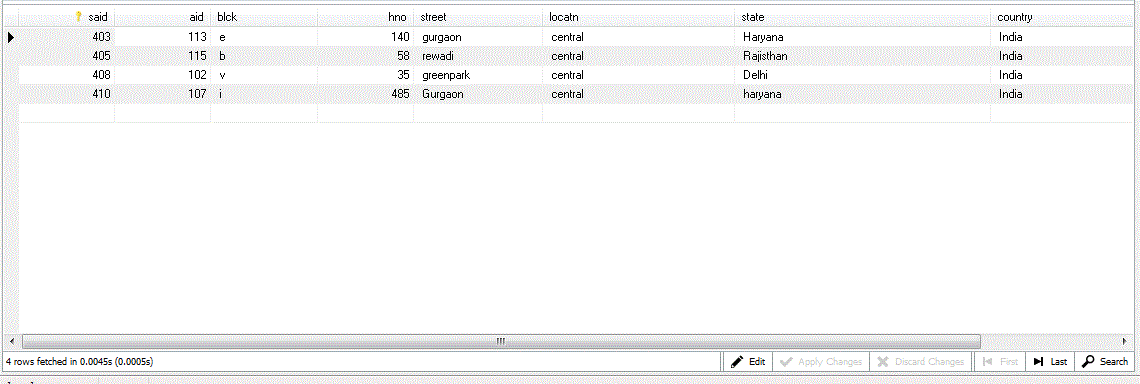### Note:

In the above code both optional value should lie in same row if they are in different row then no output will be shown as below.

### Example

```SELECT * 					//show all values
WHERE  street = 'laxminagar' 			//Both condition not satisfy
AND locatn = 'central' ;
```

### output become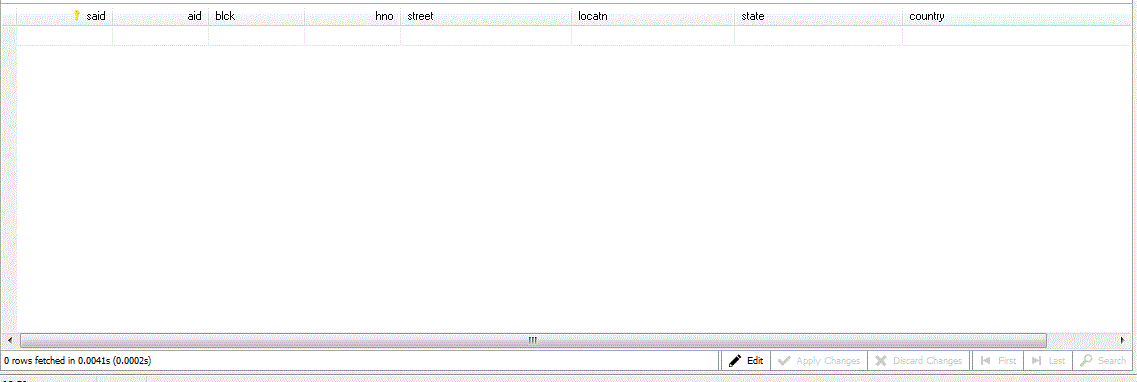### Or

This also can be used as dependently with where clause commands.

Or keyword can be used where we need to satisfy any of one condition from minimum of two conditions simultaneously within a command. Syntax of Or with Where clause is define below

• SELECT expressions
• FROM tables
• WHERE columnname optionalvalue1 or column optionalvalue2;

### Example

```SELECT *					//all values show
WHERE locatn = 'central'
OR country = 'india' ;				//OR conditions is given
```

### output become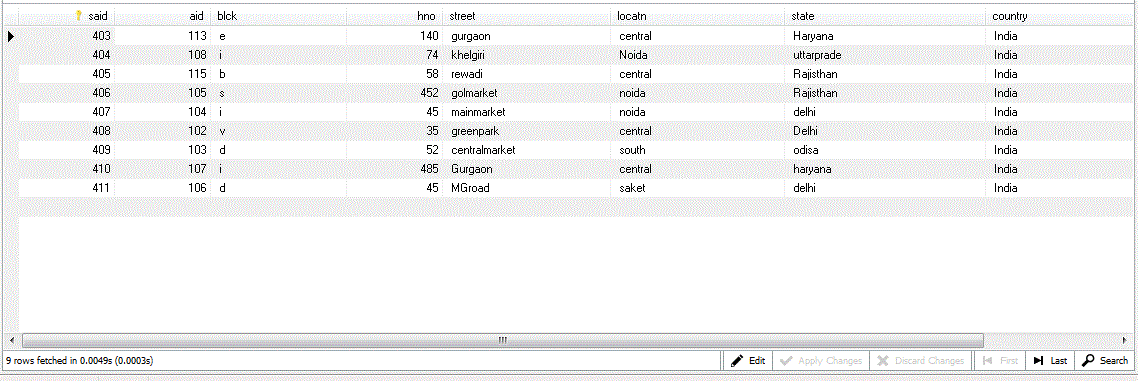### Note:

In the above example we wrote two different column value and output will print on the basis of its each condition.

### Example

Another example of different variations in conditions.

```SELECT * 					//all value show
WHERE  locatn = 'central'
OR locatn = 'saket';				//any condition need to follow
```

### output become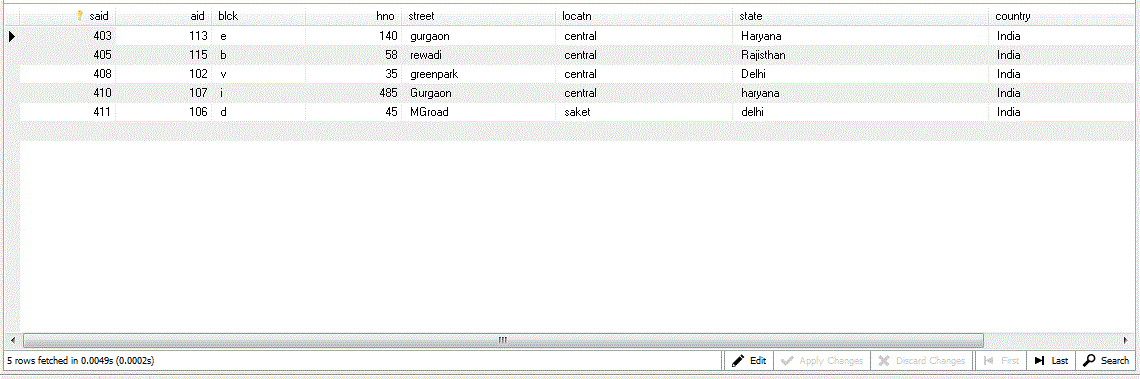### Example

```SELECT * 					//show all values
WHERE locatn='central'				//any conditions write here
OR aid > 107
```

### output become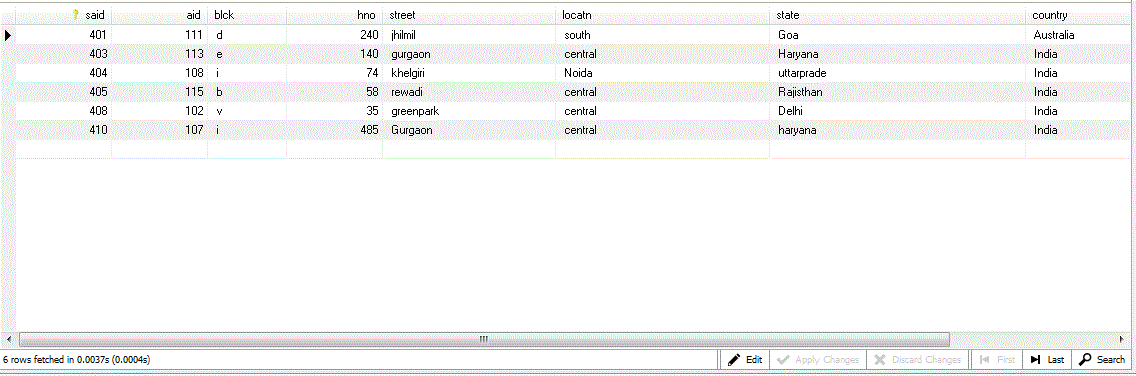### Combination of And and Or within Where clause

Here we have define some example of combination of both condition in where clause.

### Example

```SELECT *					//show all values
WHERE  locatn='central'
AND ( country ='pakistan'
OR country = 'india' );				//both condition is written here
```

### output become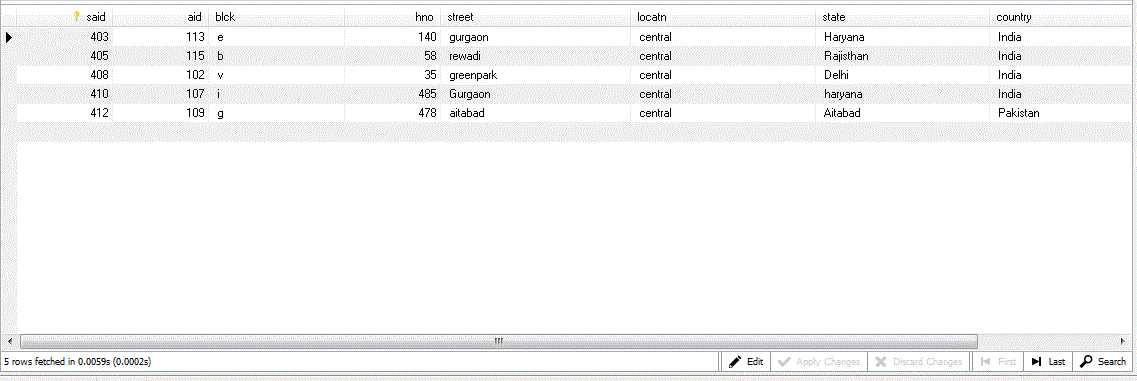### Example

Or vice-versa can be done

```SELECT *					//show all values